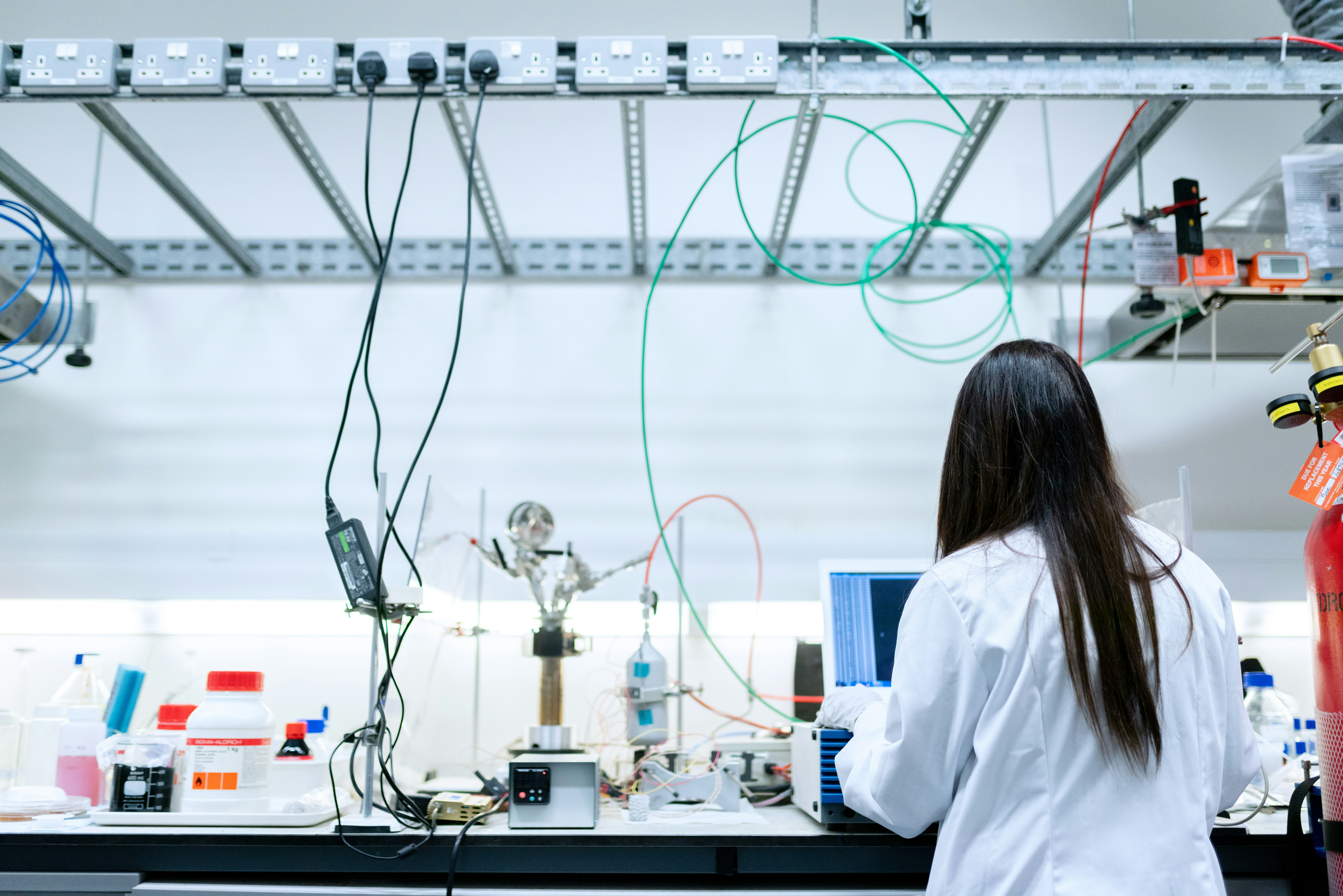Difference between Percent Yield and Theoretical Yield by@james-johnson

# Difference between Percent Yield and Theoretical Yield

June 25th 2020
3 min🖨️

Yield in chemistry is known as the total amount of product as a result of a chemical reaction. Absolute yield is weighed in terms of grams (g) and moles (mol) Theoretical yield can range in between from 0 to 100, but percentage yield can vary in ranges. Percent Yield is the percentage ratio of the product taken by a reaction. Actual yield for sodium sulfate is 37.59 g/mol and theoretical yield of 46g/4659g is 46g.### @james-johnson

James Johnson